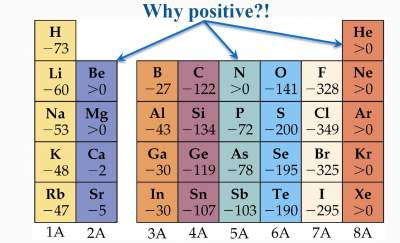# Electron Affinity

## Definition:

Electron affinity (EA)is the energy required to add an electron to an atom inThe gaseous state to form a gaseous ion

Example: Cl(g) + e → Cl (g)

Electron affinity can either be exothermic (produces heat) (as the above example) or endothermic (absorbs heat), as in this reaction:
Ar (g) + e → Ar (g)

Remark:
If EA is exothermic, it means a negative value.
If EA is endothermic, it means a positive value.

Most of the elements have negative EA; i.e exothermic, which is favourable.
Example: Cl(g) + e → Cl (g)

The extra electron in Cl adds to a subshell that has many electrons in it (3p5 ). Because of the increased repulsion forces between the new e and the existing ones, this will produce a lot of energy (exothermic)

For elements with positive EA; i.e endothermic, this is less favourable. Example: Ar(g) + e → Ar (g)

In Ar, the extra electron will be placed in the 4s orbital which is empty and higher in energy than the 3p orbital which is completely filled, i.e. minimum repulsion, but will affect the stability. This makes it unfavorable i.e. (endothermic).In N, the positive EA (endothermic) means its less favorability due to:
 7N: 1s2 2s2 2p3Based on its electronic distribution, it has a stable configuration (half filled p orbitals).
Addition of an electron disturbs this stability, hence this process is less favorable.

### Exercise on Electron Affinity

Exercise 1 on Electron Affinity

Check your answers here: Solution to the Exercise 1 on Electron Affinity

Exercise 2 on First Ionizing Energy

Check your answers here: Solution to the Exercise 2 on Electron Affinity